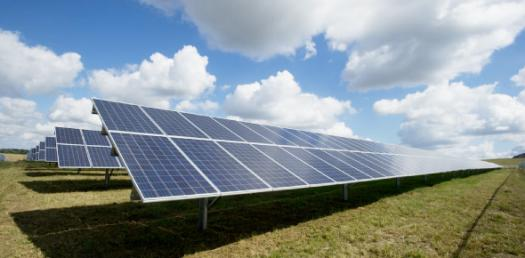# Trivia Quiz On Different Types Of Energy!

15 Questions | Total Attempts: 410SettingsTrivia Quiz on Different Types of Energy! Energy is necessarily the ability to do work, and there are different sources of energy in the world today. Some are natural, whereas others are not. In the quiz below, you get to refresh your knowledge about some of the different types of energy produced. Give it a shot and all the best!

• 1.
How can we preserve electrical energy?
• A.

Not eating

• B.

Turning off lights and tvs...... recycling

• C.

Keeping the lights on

• D.

Not recycling

• 2.
What is stored energy?
• A.

Thermal

• B.

• C.

Chemical

• D.

Potential

• 3.
What types of energy is in a battery?
• A.

Mechanical

• B.

Chemical

• C.

Thermal

• D.

Mechanical

• E.

Manget

• 4.
If a ball is being throw up in the air what two types of energy is happening.
• A.

• B.

Thermal and potentail

• C.

Kinetic and potentail

• D.

Nuclear and kinetic

• 5.
What kind of energy do stereos have?
• A.

Nuclear

• B.

Mechanical

• C.

Thermal

• D.

Sound

• E.

All of the above

• 6.
• A.

Chemical

• B.

Electrical

• C.

Solar

• D.

Sound

• E.

None of the above

• 7.
What energy do plants have?
• A.

Nuclear

• B.

Chemical

• C.

Thermal

• D.

Mechanical

• E.

None of the above

• 8.
What energy do you have when you eat?
• A.

Chemical

• B.

Sound

• C.

Kinetic

• D.

Potential

• E.

None of the above

• 9.
Using the pedals on your bike is what type of energy?
• A.

Chemical

• B.

Mechanical

• C.

Nuclear

• D.

Raidiant

• E.

None of the above

• 10.
During the day the sun gives off what?
• A.

Chemical

• B.

Raidiant

• C.

Thermal

• D.

Sound

• E.

None of the above

• 11.
When an object is in motion what type of energy dose the object have?
• A.

Kinetic

• B.

Potential

• C.

Mechanical

• D.

Electrical

• E.

Thermal

• 12.
Where does most of the earths energy come from?
• A.

Plants

• B.

Our food

• C.

The moon

• D.

The sun

• 13.
The movement of electric charges is :
• A.

Kinetic energy

• B.

Potential energy

• C.

Mechanical energy

• D.

Electrical energy

• E.

Thermal energy

• 14.
Heat is the transfer of energy.
• A.

True

• B.

False

• 15.
A _________________________ is an example of chemical energy that turns into thermal energy and gives off light and heat.
• A.

A candle

• B.

A fan

• C.

A tv

• D.

A glass of water

Related TopicsBack to top